# 一类三阶半线性中立型时滞微分方程的振动性Oscillation of a Class of Third Order Semi-Linear Neutral Delay Differential Equations

• 全文下载: PDF(463KB)    PP.473-480   DOI: 10.12677/AAM.2019.83053
• 下载量: 118  浏览量: 177   科研立项经费支持

The oscillations of a class of neutral third order semi-linear differential equations are studied. Different functions and classical inequalities are constructed by using Riccati transformation techniques. Some new oscillatory theories of this kind of differential equations are established. The conclusions generalize and improve the relevant results in the literature, and illustrate the application of the new oscillatory conclusions with examples.

1. 引言

${\left[r\left(t\right){|{Z}^{″}\left(t\right)|}^{\alpha -1}{Z}^{″}\left(t\right)\right]}^{\prime }+q\left(t\right){|x\left(\sigma \left(t\right)\right)|}^{\beta -1}x\left(\sigma \left(t\right)\right)=0,\text{\hspace{0.17em}}t\ge {t}_{0}>0,\text{\hspace{0.17em}}\beta >\alpha .$ (E)

(A1) $p\left(t\right),q\left(t\right)\in C\left(\left[{t}_{0},\infty \right),\left(0,\infty \right)\right),0\le p\left(t\right)\le p<1,q\left(t\right)>0$

(A2) $r\left(t\right)\in {C}^{1}\left(\left[{t}_{0},\infty \right),\left(0,\infty \right)\right),r\left(t\right)\ge 0,{r}^{\prime }\left(t\right)\ge 0,{\int }_{{t}_{\text{0}}}^{\infty }{r}^{-\frac{1}{\alpha }}\left(s\right)\text{d}s\le +\infty$

(A3) $\tau \left(t\right),\sigma \left(t\right)\in {C}^{1}\left(\left[{t}_{0},\infty \right),\left(0,\infty \right)\right)$ ，对每一 $t\ge {t}_{0}$ ，都有 $\tau \left(t\right)\le t,\sigma \left(t\right)\le t$

$\sigma \left(t\right)>0,{\sigma }^{\prime }\left(t\right)>0,\underset{t\to \infty }{\mathrm{lim}}\tau \left(t\right)=\underset{t\to \infty }{\mathrm{lim}}\sigma \left(t\right)=\infty .$

${\left(r\left(t\right){|{Z}^{\prime }\left(t\right)|}^{\alpha -1}{Z}^{\prime }\left(t\right)\right)}^{\prime }+q\left(t\right){|x\left(\sigma \left(t\right)\right)|}^{\beta -1}x\left(\sigma \left(t\right)\right)=0.$ (1.1)

${\int }_{{t}_{0}}^{\infty }{r}^{-\frac{1}{\alpha }}\left(s\right)\text{d}s\le +\infty$$\beta >\alpha \text{ },\beta <\alpha ,\beta =\alpha$ 的考虑 $\beta >\alpha$ 。情形新的振动性结论，我们的结果推广和改进了

2. 引理

$\left(A\right)\text{ }\text{ }\text{ }\text{ }\text{ }\text{ }\text{ }\text{ }Z\left(t\right)>0,{Z}^{\prime }\left(t\right)>0,{Z}^{″}\left(t\right)>0.$

$\left(B\right)\text{ }\text{ }\text{ }\text{ }\text{ }\text{ }\text{ }\text{ }Z\left(t\right)>0,{Z}^{\prime }\left(t\right)<0,{Z}^{″}\left(t\right)>0.$

$u\left(\sigma \left(t\right)\right)\ge \theta \frac{\sigma \left(t\right)}{t}u\left(t\right),\text{ }\text{ }\text{ }\text{ }\text{ }\text{ }\text{ }\text{ }\text{ }\text{ }t\ge {T}_{\theta }$

$u\left(t\right)\ge \gamma t{u}^{\prime }\left(t\right)\text{ },\text{ }\text{ }\text{ }\text{ }\text{ }\text{ }\text{ }\text{ }t\ge {T}_{\gamma }$

$\underset{t\to \infty }{\mathrm{lim}}{\int }_{{t}_{0}}^{t}{\int }_{u}^{\infty }{\left(\frac{1}{r\left(v\right)}{\int }_{v}^{\infty }q\left(s\right)\text{d}s\right)}^{\frac{1}{\alpha }}\text{d}v\text{d}u=+\infty .$ (2.1)

$\underset{t\to +\infty }{\mathrm{lim}}x\left(t\right)=0$

3. 主要结果

$D=\left\{\left(t,s\right)|t\ge s\ge {t}_{0}\right\}$${D}_{0}=\left\{\left(t,s\right)|t>s\ge {t}_{0}\right\}$

i)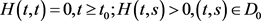ii)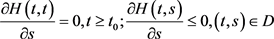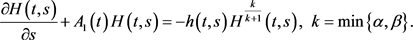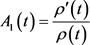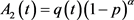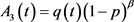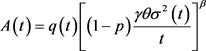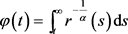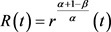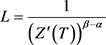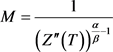，T充分大。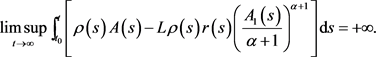(3.1)为(A)型，即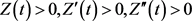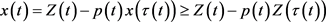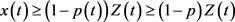(3.2)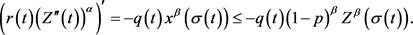(3.3)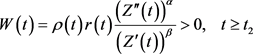(3.4)

(3.4)式两边对t进行求导，并利用(3.3)，(3.4)得(3.5)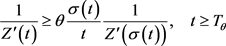(3.6)(3.7)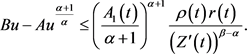(3.8)，由于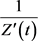单调递减，所以当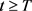时，有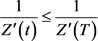。又因为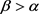，于是有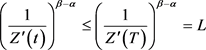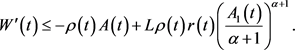(3.9)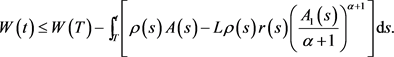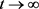，根据(3.1)式，则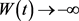，这与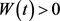矛盾，故假设不成立，即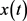是方程(E)的振动解。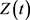满足(B)型，由于(2.1)式成立，故由引理2.5可得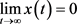。证毕。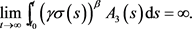(3.10)(3.11)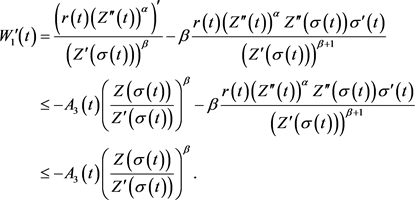(3.12)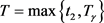，使当时，(3.12)式变成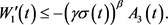(3.13)，则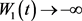，这与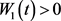矛盾，故假设不成立，即是方程(E)的振动解。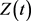满足(B)型，由于(2.1)式成立，故由引理2.5可得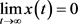。证毕。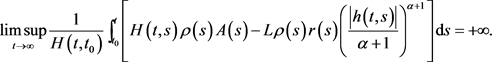(3.14)(3.15)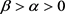，故有(3.16)满足(B)型，由于(2. 1)式成立，故由引理2.5可得。证毕。

4. 应用(E1)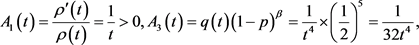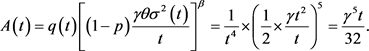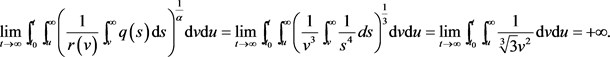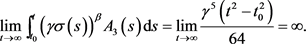1) 广东省茂名市科技计划项目(2015038)；2) 广东石油化工学院理学院科研扶持基金重点项目(KY2018001)。

NOTES

*通讯作者。

  李元旦, 高正晖, 邓义华. 三阶半线性中立型微分方程的振动性[J]. 北华大学学报(自然科学版), 2012, 13(3): 267-270.  苏新晓, 戴丽娜, 伍思敏, 林全文. 二阶半线性中立型微分方程的振动性[J]. 应用数学进展, 2017, 6(3): 417-422.  Liu, H., Meng, F. and Liu, P. (2012) Oscillation and Asymptotic Analysis on a New Generalized Emden-Fowler Equation. Applied Mathematics and Computation, 219, 2739-2748. https://doi.org/10.1016/j.amc.2012.08.106  曾云辉, 罗李平, 俞元洪. 中立型Emden-Fowler时滞微分方程的振动性[J]. 数学物理学报, 2015, 35A(4): 803-814.  罗李平, 俞元洪, 罗振国. 三阶非线性中立型微分方程的振动分析[J]. 系统科学与数学, 2016, 36(4): 551-559.  林文贤. 三阶半线性中立型阻尼泛函微分方程的振动性[J]. 华东师范大学学报(自然科学版), 2017(3): 48-53.  Dzurina, J. and Baculikova, B. (2012) Oscillation of Third-Order Quasi-Linear Advanced Differential Equations. Differential Equations & Applications, 4, 411-421. https://doi.org/10.7153/dea-04-23  李同兴, 韩振来, 张承慧, 等. 时间尺度上三阶Emden-Fowlwe时滞微分方程的振动准则[J]. 数学物理学报, 2012, 32(1): 222-232.  曾云辉, 俞元洪. 三阶半线性时滞微分方程的振动定理[J]. 系统科学与数学, 2014, 34(2): 231-237.  Qin, G., Huang, C., Xie, Y., et al. (2013) Asymptotic Behavior for Third-Order Quasi-Linear Differential Equations. Advances in Difference Equations, 2013, 305. https://doi.org/10.1186/1687-1847-2013-305  仉志余, 王晓霞, 俞元洪. 三阶半线性中立型分布时滞微分方程的振动性[J]. 应用数学学报, 2015, 38(3): 450-459.  李全娣, 杨菊, 黎小贤, 林全文. 一类三阶中立型半线性时滞微分方程振动准则[J]. 理论数学, 2017, 7(4): 356-362.  林全文, 俞元洪. 三阶半线性时滞微分方程的振动性和渐进性[J]. 系统科学与数学, 2015, 35(2): 233-244.  惠远先, 王俊杰. 三阶中立型半线性时滞微分方程的振动性[J]. 井冈山大学学报, 2017, 38(1): 8-13.  Hardy, G., Litterwood, J. and Polya, G. (1952) Inequalities. 2nd Edition, Cambridge University Press, Cambridge.  Erbe, L. (1973) Oscillation Criteria for Second Order Nonlinear Delay Equations. Canadian Mathematical Bulletin, 16, 49-56. https://doi.org/10.4153/CMB-1973-011-1